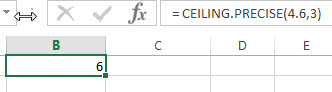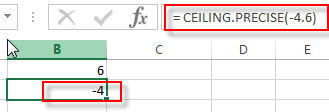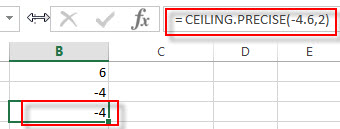# Excel CEILING.PRECISE Function

This post will guide you how to use Excel CEILING.PRECISE function with syntax and examples in Microsoft excel.

### Description

The Excel CEILING.PRECISE function returns a given number rounded up to the nearest multiple of a given number of significance or returns a number that is rounded up to the nearest integer number.

The CEILING.PRECISE function is a build-in function in Microsoft Excel and it is categorized as a Math and Trigonometry Function.

The CEILING.PRECISE function is available in Excel 2016, Excel 2013, Excel 2010, Excel 2007, Excel 2003, Excel XP, Excel 2000, Excel 2011 for Mac.

### Syntax

The syntax of the CEILING.PRECISE function is as below:

`=CEILING.PRECISE (number, [significance])`

Where the CEILING.PRECISE function arguments are:

• number – This is a required argument. The number that you want to round up.
• significance – This is an optional argument. The multiple of significance to which you want to round a number to. If the significance argument is omitted, the default value will be set to 1.

Note:

• If either number or significance arguments are non-numeric, the CEILING.PRECISE function will return the #VALUE! Error.
• Regardless of the sign of the number, the number is rounded up.
• If the number or the significance argument is zero, the CEILING.PRECISE function will return zero.

### Excel CEILING.PRECISE Function Examples

The below examples will show you how to use Excel CEILING.PRECISE Function to round a number up to nearest multiple.

1# to round 4.6 up to nearest multiple of 3, enter the following formula in Cell B1.

= CEILING.PRECISE(4.6,3)2# to round -4.6 up to nearest multiple of 1, enter the following formula in Cell B2.

= CEILING.PRECISE(-4.6)3# to round -4.6 up to nearest multiple of 2, enter the following formula in Cell B3.

= CEILING.PRECISE(-4.6,2)### Related Functions

• Excel ROUNDDOWN function
The Excel ROUNDDOWN function round the number down to the specified number of digits. The syntax of the ROUNDDOWN function is as below:=ROUNDDOWN (number, num_digits)…
• Excel ROUND function
The Excel ROUND function rounds a number to a specified number of digits. You can use the ROUND function to round to the left or right of the decimal point in Excel.The syntax of the ROUND function is as below:=ROUND (number, num_digits)…
• Excel ROUNDUP function
The Excel ROUNDUP function rounds the number up to a specified number of decimal places. It will round away from 0.The syntax of the ROUNDUP function is as below:=ROUNDUP (number, num_digits)…
• Excel CEILING Function
The Excel CEILING function returns a given number rounded up to the nearest multiple of a given number of significance. The syntax of the CEILING function is as below:= CEILING (number, significance)…